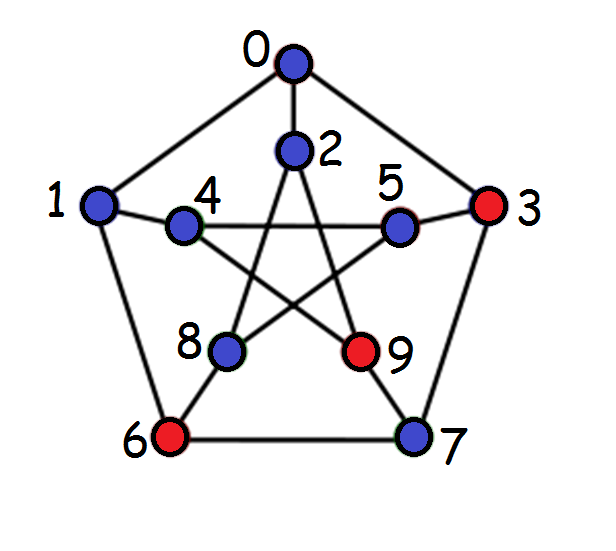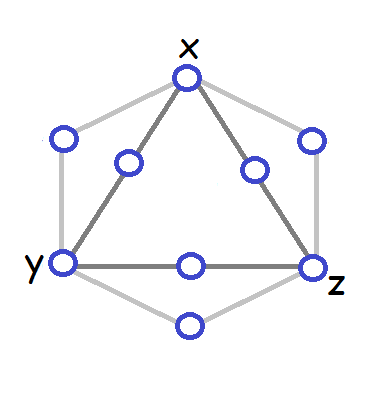### CSC 320: Summer 2017 Assignment #5, Due at beginning of class, Fri. July 28, 2017

Instructions for all assignments: Draw boxes for your marks on the top of the first page of your submission. Place a 0 in the corresponding box for any questions you omit. For this assignment:
Question12345 6 7 8
Marks 0 0 0 0 0 0 0 0

1.  A TM M with start state s decides a language L if
(s, # w [#]) |-* (h, # Y [#]) for w in L and
(s, # w [#]) |-* (h, # N [#]) for w not in L.
Suppose you have three TM's M1, M2, and M3 that respectively decide the three languages L1, L2, and L3 that are defined over the alphabet {a,b}. Give the machine schema for a TM M that decides the language L= { w in {a,b}* : w is in at least two of the languages L1, L2 or L3}. You can use a copy machine C in your machine schema. The copy machine C when started with (s, # w [#]) for a string w over {a,b} halts with (h, # w # w [#]).
2. The aim of this question is to prove that it is possible to design an algorithm that takes as input a TM M and an input string w that can determine whether or not M ever moves it head to the left when computing on the input string w.
(a)  Design a TM M that has alphabet {#, a, b} and four states {s, t, u, v} that takes AS MANY STEPS AS POSSIBLE before an observer watching the computation of M on input bab who can see the steps of the computation but not the description of your TM (and does not know the number of states/symbols it has) can conclude that the TM is never going to move its head to the left. Hand in the output you get from running the TM simulator on your TM edited to stop at the point where the observer can tell that the TM is not going to ever move its head to the left. Explain why it is obvious that the TM M will never move its head to the left at that point.

If you save your TM in q2.txt, to make output to print, run the TM simulator like this:
javac *.java
java TM < q2.txt > oq2.txt
The TM simulator has infinite loop protection in it to ensure that it will not continue computing forever. If you do not want to lose marks for this question, make sure that you EDIT oq2.txt before printing it to eliminate the steps that occur after the observer can conclude that the TM never moves its head to the left.
(b)  The TM simulator starts numbering the initial configuration with Step 0. For example, with start state s and input bab, it starts with:
Step 0 : (s, #bab[#])
For an arbitrary TM M and input string w, what is the maximum Step Number that the TM simulator would have to print before you you can determine from watching the steps of the computation of M on input w either that it moves its head to the left or that it never will? Express your answer as a function of k (the number of states of the TM M) and s (the number of tape symbols in M's alphabet). Justify your answer.
(c)  Consider the following question:
Given a TM M, and input w, does M ever move its head to the left when started in a configuration (s, # w [#]), where s is the start state of M?
How could you modify my TM simulator program to turn it into a program that can decide the answer to this question? Hint: Use your answer to (b).

3. Suppose you are given two TM's M1 and M2 that accept languages L1 and L2 respectively. On a previous final exam, I asked students to give a high-level description of a TM M that halts on input aa if aa is in L1 union L2 and otherwise, it does not halt. One student provided this solution:
Run M1 on aa and if it halts then halt with the answer yes. Otherwise, run M2 on input aa
(a)  Provide the machine schema for two specific TM's M1 and M2 such that this approach will prove ineffective.
(b)  How can you modify the student answer to provide a correct solution to the final exam question?
(c)  What could you do to design a TM that halts if and only if there is some string over {a}* such that both M1 and M2 halt on this string?
4. Suppose the head instructions for a TM M are numbered as follows:
 0 1 2 3 4 5 6 7 8 9 L R # ( ) q a 0 1 ,

The string "M" is:
(q01, a0010, q01, a0000), (q01, a0100, q01, a0000), (q01, a0101, q00, a0101), (q01, a0111, q01, a0000), (q01, a1000, q10, a0001), (q10, a0111, q10, a0000), (q10, a1000, q10, a0001), (q10, a0101, q00, a1000)

(a)  What is M?
Start state of M:
 State Symbol Next state Head Instruction ______ ______ ___________ _________________ ______ ______ ___________ _________________ ______ ______ ___________ _________________ ______ ______ ___________ _________________ ______ ______ ___________ _________________ ______ ______ ___________ _________________ ______ ______ ___________ _________________ ______ ______ ___________ _________________

(b)  Is the question:
Does M from part (a) halt on input "M"?
decidable or not? You can assume that the problem:
Given M, does M halt when started on a blank tape?

5. Consider these two problems:
Problem P1: Given a TM M, does M halt on every input string w in {a,b}* that has length 3 or less?
Problem P2: Given a TM M, does M halt on input string abaab?

(a)  Prove that if you have an algorithm for problem P1 then you can design an algorithm that finds the answer to problem P2.
(b)  Which one of these statements does your answer to (a) prove:
S1: If P1 is not decidable them P2 is not decidable.
S2: If P2 is not decidable them P1 is not decidable.

6. A subset S of the vertices of a graph G is called a Dominating Set if every vertex v of G is either in S or v has a neighbour which is in S (that is, there is some vertex u in S such that (u,v) is an edge of G).
(a)  Describe in terms of the input and output the (hard) variant of this optimization problem which is in NP.
Input:
Output:
(b)  Describe precisely a certificate for this problem that could be typed in as input using only integer values.
(c)  Show what you would type in as a certificate for the dominating set (the red vertices) for this graph:(d)  Give detailed pseudocode for an efficient algorithm for reading in your certificate and checking if it is valid. Assume that the graph G is already stored using an adjacency list representation.
(e)  Provide a tight analysis of the time complexity (use THETA notation) for your approach from (d) for the case that the input graph is stored in an adjacency list representation. Justify your answer by annotating your algorithm from (d) to indicate the time complexity of each step of your certificate checking algorithm.

7. (a) Given a 3-SAT problem, construct a graph as follows: For each variable x, the graph contains a triangle as pictured here:For each clause, there is a vertex of the graph connected to the three literals in the clause. If the 3-SAT problem has k variables and c clauses then this constructed graph has 3k+c vertices and 3k+3c edges.
(a)  Draw the graph that arises from this 3-SAT system:(b)  Find a satisfying assignment of this 3-SAT system and show a corresponding dominating set on the graph.
(c)  For a general problem, if the 3-SAT system has a satisfying truth assignment, what size dominating set can you find?
(d)  Prove that it is not possible to find a dominating set of the size you specified for part (c) when the 3-SAT system has no satisfying truth assignments.
8. [Bonus question] Suppose that instead of using a single vertex for each clause of the 3-SAT system, a gadget like this is used instead:where the variables x, y, and z in the clause gadget are connected to the corresponding triangle vertices as per the previous question.
(a)  What is the minimum size of a dominating set that the resulting graph will have when the 3-SAT problem is solvable and what will a minimum dominating set look like? Justify your answer.
(b)  Prove that if the 3-SAT problem has no solutions then the graph does not have a dominating set of the size you indicated for part (a).